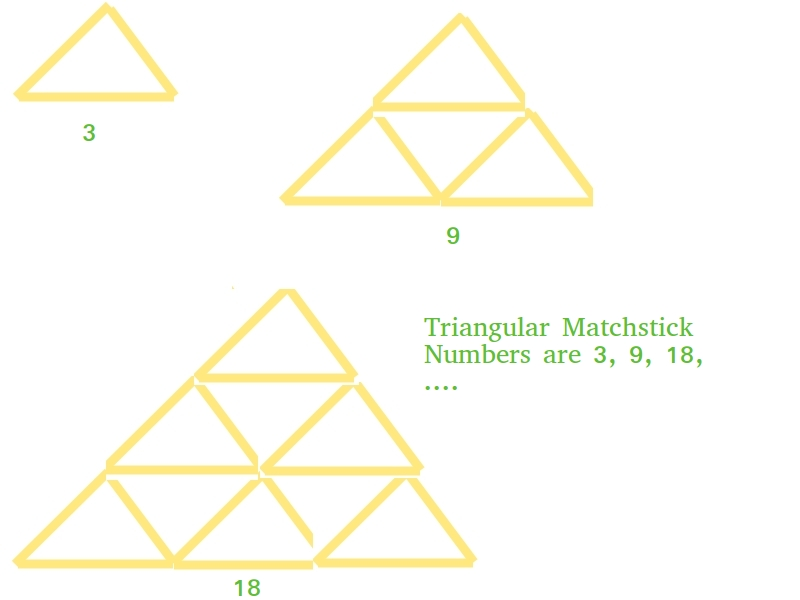Python Program for Triangular Matchstick Number

python tutorials and learn python

Python Program for Triangular Matchstick Number

Given a number X which represents the floor of a matchstick pyramid, write a program to print the total number of matchstick required to form a pyramid of matchsticks of x floors.

Examples:

Input : X = 1
Output : 3
Input : X = 2
Output : 9

This is mainly an extension of triangular numbers. For a number X, the matchstick required will be three times of X-th triangular numbers, i.e., (3*X*(X+1))/2# Python program to find X-th triangular # matchstick number  def numberOfSticks(x):     return (3 * x * (x + 1)) / 2     # main() print(int(numberOfSticks(7)))
Output:

84